# Inserting LaTEX mathematical formulae

[SPIP 1.8] introduces a new powerful functionality that allows complex mathematical formulae to put inserted into text using the syntax defined for TEX/LaTEX.

For example, this functionality can allow you to show formulae like this one: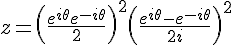by entering the relevant code directly into the text, just as one would with TEX.

Important note: using this method naturally requires familiarity with the formula syntax of TEX. A syntax which is not exactly straightforward... 

## Images within the text

The technical base of this method consists of converting each of the formulas into an image, an image which is subsequently displayed within the text. At the time of writing, this is the simplest and most effective means of displaying complex mathematical formulae on a web page.

At the moment, the display of mathematical formulae on web pages using the MathML standard is not always feasible, chiefly because the implementations of MathML in browsers are widely divergent and often unreliable. The solution supported by SPIP (integrating images representing the formulae) is currently the only one that can guarantee that all site visitors will be able to see the mathematical formulae correctly.

It is important to understand that in SPIP, only formulae can be converted. It is out of the question, in particular, to use any of the macro functions of TEX to affect the presentation of the page document. What is simply provided here is a tool that permits you to integrate mathematical formulae within the SPIP code as is normally performed for other SPIP objects.

## SPIP-specific syntax

The SPIP syntax required consists of nesting the relevant text segment within pseudo-tags as demonstrated below:

$... Put your mathematical formulae here... ...$

Whenever your text consists of only mathematical formulae, you can naturally apply the pseudo-tags $...$ on a global level (that is: put $ right at the start of the text, and $ right at the end...).

The only known incompatibility here occurs when you wish to put the "dollar" ($) symbol in the text, as the "dollar" symbol is used as a formula delimiter. (In reality, that is why the pseudo-codes  exist at all, to indicate that the dollar symbol will act as a pre-processor flag for the contained text segment) Within these pseudo-tags, you then need only code mathematical formulae according to the syntax of TEX, by delimiting each formula with dollars ($) or with double-dollars () for a centred formula.

Here is an example:

You can insert matrices: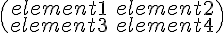you can define complex fractions such as: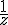,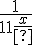you can use greek letters: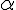,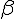,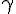,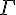,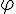present a system of equations: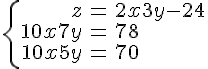and show complex formulae as centred text: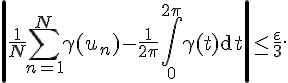which is coded as below in the original text:

$You can insert matrices: \begin{pmatrix}element1&element2 \\element3&element4\end{pmatrix} _ you can define complex fractions such as: {1\over z}, {1\over\displaystyle 1+{1\over x}} _ you can use greek letters: \alpha, \beta, \gamma, \Gamma, \varphi _ present a system of equations: \left\{\begin{array}{rcl} z&=&2x+3y-24\\10x+7y&=&78\\10x+5y&=&70\\\end{array}\right _ and show complex formulae as centred text: \left|{1\over N}\sum_{n=1}^N \gamma(u_n)-{1\over 2\pi}\int_0^{2\pi}\gamma(t){\rm d}t\right| \le {\varepsilon\over 3}.$

This system is limited to displaying well-formed mathematical formulae and no other TEX functionality is available. In particular: it is not possible to define your own macros (\def...{...} is not available) and macros other than anything specifically a mathematical formula will not be recognised. In practical usage, other limitations will be encountered, but it is important to recall that the goal of this feature is ONLY to show mathematical formulae within text, and nothing more...

## For webmasters

Equations are treated as in client-server mode: formulae are sent to the server, which returns graphical image files of each equation. (These files are, naturally, cached on your site, and this process of transfer occurs only once for each equation.)

For further information on the topic, please refer to the SPIP Wiki page about LaTeX and LaTeX servers. There is information there which provides instructions on how to implement your own formula server, and no longer rely on our centralised server.

Footnotes

[1wikipedia : Help:Displaying_a_formula

Author Published : Updated : 10/09/22

Translations : català, corsu, English, Español, français, italiano, Nederlands, Português do Brasil, slovenčina, українська